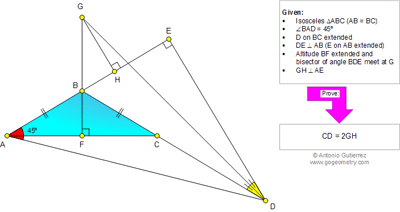## Thursday, January 16, 2014

### Geometry Problem 958. Isosceles Triangle, Altitude, 45 Degrees, Perpendicular, Angle Bisector, Metric Relations

Geometry Problem
Level: Mathematics Education, High School, Honors Geometry, College.

Click the figure below to see the complete problem 958.#### 1 comment:

1.Note that G is the center of excircle of triangle BDE
Radius of excircle Rd= GH= p-ED .. where p= half of perimeter
2p=ED+EB+BD
p-ED=1/2(EB+BD-ED) … (1)
Since ADE is right isosceles triangle => ED=EA
(1) become GH= ½(EB+BD-EA)=1/2(BD-BA)=1/2(BD-BC)=1/2CD